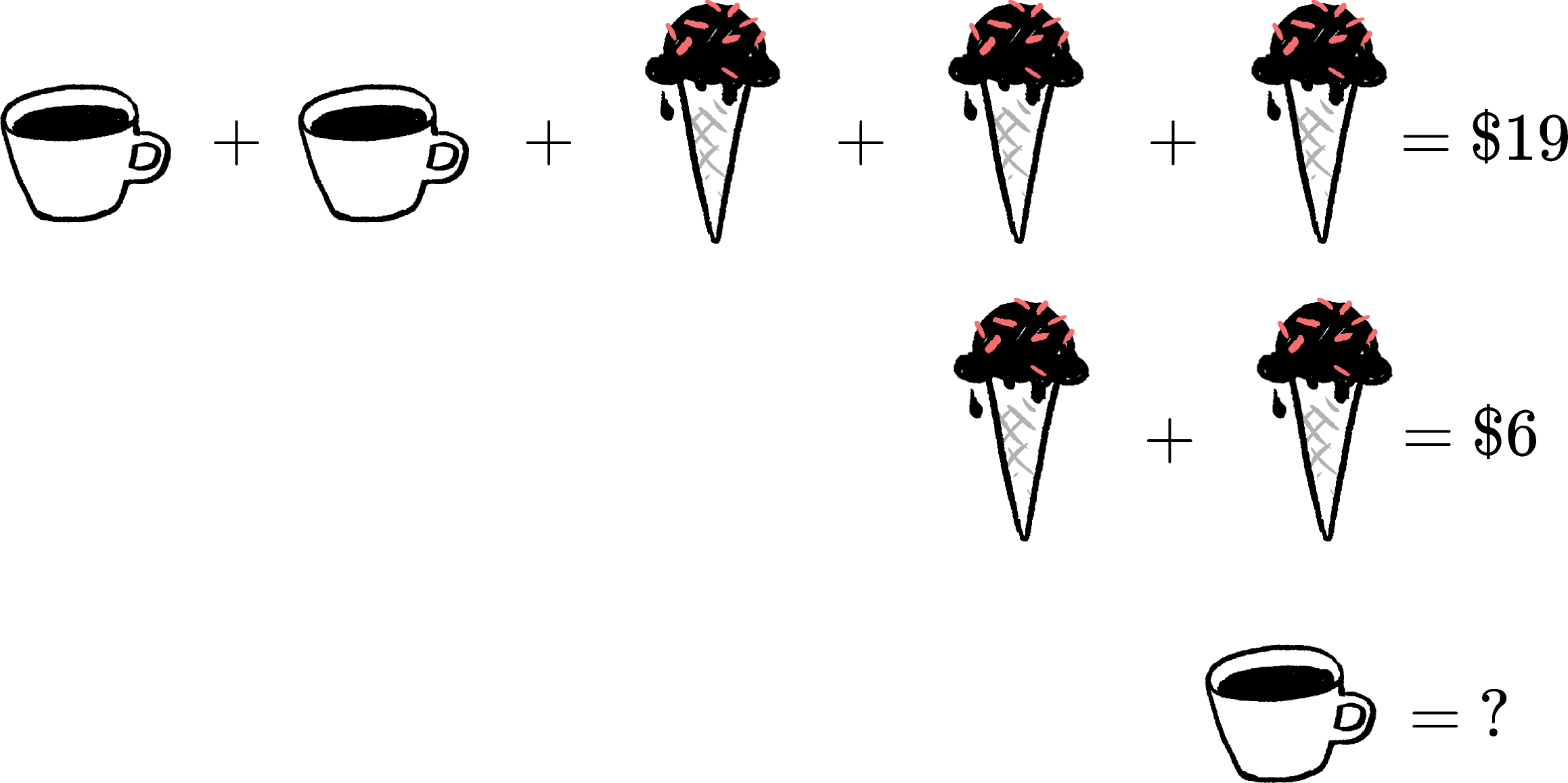### Pre-AlgebraWhat is the price at the question mark?

# Starting With VariablesThe symbols above are direct references to what they mean, but we can use any symbols we like. If $C$ is the cost of a cup of cocoa and $I$ is the cost of one cone of ice cream, the first two lines above are equivalent to

\begin{aligned} C + C + I + I + I &= 19 \\ I + I &= 6 \end{aligned}

$C$ and $I$ here are both variables, values that can vary and are only fixed to set numbers in order to fulfill the specific conditions of the problem.

# Starting With Variables

Micah has $\20.$ Sara has $\4.$ If Micah gives some money to Sara, how much does Micah need to give so at the end they both have the same amount of money?

# Starting With Variables

The prior problem could be represented with the variable $a$ being the amount of money given:

• Micah starts with $20$ and loses $a,$ so she has $20 - a$ at the end
• Sara starts with $4$ and gains $a,$ so she has $4 + a$ at the end

In other words, the problem was to find $a$ such that

$20 - a = 4 + a$

# Starting With Variables

Three squares each contain an identical number of dots.

How many dots should go in each square so that the squares have a combined total of $21$ dots?

# Starting With Variables

The count of dots per square is acting as a variable. Not only is it an amount that can vary but it can also be reused, and each instance of the variable represents the same number of dots.

With letters, we could assign the number of dots as $D,$ and the previous question is asking when

$D + D + D$

equals $21.$

We can also simplify $D + D + D$ into $3 \times D ,$ which is considered equivalent.

×